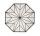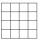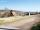Areaf of ST

It is given square DBLK with side |BL|=13. Calculate area of triangle DKU if vertex U lie on line LB.

Result

S =  84.5

Solution:Leave us a comment of example and its solution (i.e. if it is still somewhat unclear...):Be the first to comment!Next similar examples:

1. Triangle ABCConstruct a triangle ABC is is given c = 60mm hc = 40 mm and b = 48 mm analysis procedure steps construction
2. Octagonal matOctagonal mat formed from a square plate with a side of 40 cm so that every corner cut the isosceles triangle with leg 3.6 cm. What is the content area of one mat?
3. SquareSquare JKLM has sides of length 24 cm. Point S is the center of LM. Calculate the area of the quadrant JKSM in cm2.
4. Trapezium 2Trapezium has an area of 24 square cms. How many different trapeziums can be formed ?
5. Right triangleRight triangle ABC with side a = 19 and the area S = 95. Calculate the length of the remaining sides.
6. Parcelparcel has a rectangular shape of a trapezoid with bases 12 m and 10 m and a height 8 m. On parcel was built object with a footprint an isosceles triangle shape with side 4 m and height three-quarters of a meter. What is the area of unbuild parcel?
7. Area of ditchHow great content area will have a section of trapezoidal ditch with a width of 1.6 meters above and below 0.57 meters? The depth of the ditch is 2.08 meters.
8. GlovesI have a box with two hundred pieces of gloves in total, split into ten parcels of twenty pieces, and I sell three parcels. What percent of the total amount I sold?
9. AcreagePlot has a diamond shape, its side is 25.6 m long and the distance of the opposite sides is 22.2 meters. Calculate its acreage.
10. TVsProduction of television sets increased from 3,500 units to 4,200 units. Calculate the percentage of production increase.
11. Center traverseIt is true that the middle traverse bisects the triangle?
12. NumberWhat number is 20 % smaller than the number 198?
13. InequationSolve the inequation: 5k - (7k - 1)≤ 2/5 . (5-k)-2
14. Down syndromeDown syndrome is one of the serious diseases caused by a gene mutation. Down syndrome occurs in approximately every 550-born child. Express the incidence of Down's syndrome in newborns at per mille.
15. Simplify 2Simplify expression: 5ab-7+3ba-9
16. EquationSolve the equation: 1/2-2/8 = 1/10; Write the result as a decimal number.
17. MO 2016 Numerical axisCat's school use a special numerical axis. The distance between the numbers 1 and 2 is 1 cm, the distance between the numbers 2 and 3 is 3 cm, between the numbers 3 and 4 is 5 cm and so on, the distance between the next pair of natural numbers is always in